# Identify Graphs of Functions - Tutorials

This is a tutorial on identifying the graph of an algebraic function given by its formula. Four graphs are given and only one of them is the possible graph corresponding to the given function. Examples with detailed solutions are presented.

SEARCH THIS SITEWeb www.analyzemath.com

You may want to go through tutorials on graphing before starting this tutorial.

Example 1 : Identify the graph of function    f(x) = -x2 - 1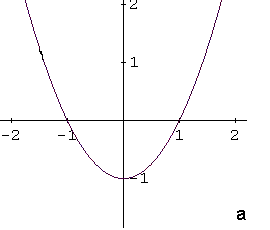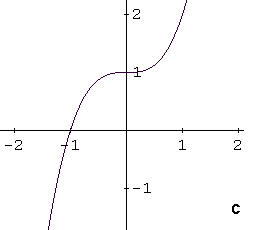Solution to Example 1:

The given function f(x) = -x2 - 1 is a quadratic one and its graph is a parabola. Writing function f in the form f(x) = a(x - h)2 + k   makes it easy to graph. The x and y coordinates of the  vertex are given respectively by h and k. When coefficient a is positive the parabola opens upward. If a is negative the parabola opens downward.

The given function f can be written as f(x) = -(x - 0)2 - 1. Its vertex has x coordinate equal to 0 and y coordinate equal to -1. Its coefficient a is negative and the parabola opens downward. Also its y intercept  is at (0 , -1).

The above properties of the graph of function f shows that the graph corresponding to the given equation is graph d.

Example 2 : Identify the graph of function    f(x) = |x - 1| + 1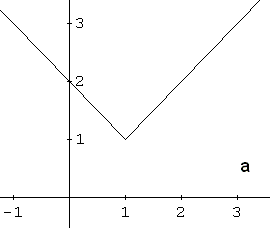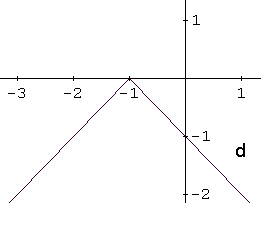Solution to Example 2:

The given function f(x) = |x - 1| + 1 is related to the absolute value function |x|. We now use a different strategy from the one we used in example 1. The graph of |x - 1| is the graph of |x| that has been shifted one unit to the right as shown below.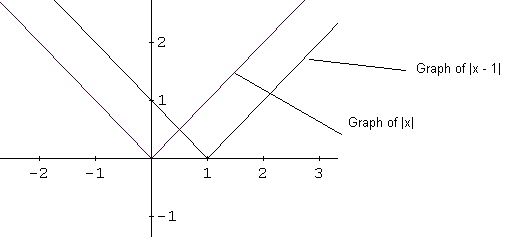The graph of |x - 1| + 1 is the graph of |x - 1| shifted one unit up as shown below.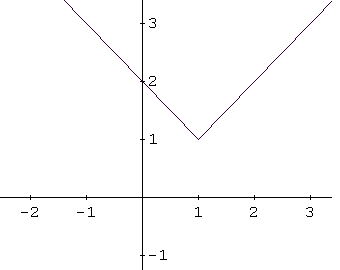The above graph correspond to graph a in example 2.

Now that you have worked on the above examples you may go to self test on graphs of functions and solve similar questions.